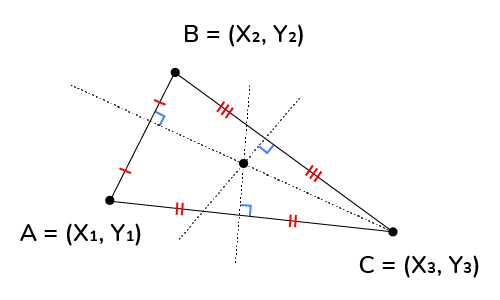# Circumcenter Calculator

Created by Anna Szczepanek, PhD
Reviewed by Davide Borchia
Last updated: Jun 08, 2022

Our circumcenter calculator will gently introduce you to the important topic of determining a triangle's circumcenter given the coordinates of vertices. Scroll down to learn more about:

• What the circumcenter of a triangle is all about;
• How to use this circumcenter calculator most efficiently;
• What fascinating properties the circumcenter has;
• How to construct the circumcenter using compass and ruler; and
• What the formula for the circumcenter of a triangle given vertices looks like.

## What is the circumcenter of a triangle?

The circumcenter of a triangle is the center of the triangle's circumscribed circle (circumcircle), that is, the circle that passes through all the vertices of the triangle. We can quite easily determine the circumcenter as the point where the triangle's perpendicular bisectors intersect.

#### Properties of the circumcenter

• The most important property of the circumcenter is the following: The distance between the circumcenter and each of the triangle's vertices is the same. In other words, the circumcenter is equidistant from all three vertices.

• Another very useful property says that the location of the circumcenter depends on the type of triangle we're dealing with:

• If the triangle is acute, the circumcenter lies inside the triangle.
• If the triangle is right, the circumcenter coincides with the midpoint of the hypotenuse.
• If the triangle is obtuse, the circumcenter lies outside of the triangle.
• The circumcentre of a triangle coincides with the orthocenter of its medial triangle, i.e., the triangle whose vertices are located at the midpoints of the sides of the original triangle.

We'll get back to these properties while discussing how to find the circumcenter of a triangle!

## How to use this circumcenter calculator?

Using our circumcenter calculator is not complicated at all: all you have to do is to input the coordinates of each vertex of your triangle in the appropriate fields. The coordinates of the circumcenter will immediately appear at the bottom of our tool!

Are you wondering how our circumcenter calculator works? What circumcenter formula does it use to solve the problem so quickly? We'll reveal this secret in the next section.

## How to find the circumcenter of a triangle? Circumcenter formula

Here we'll show you how to derive the formula for the coordinates of the circumcenter given the coordinates of the triangle's vertices.

Let $(x_1, y_1), (x_2, y_2), (x_3, y_3)$ stand for these coordinates. The (unknown) coordinates of the circumcentre will be denoted by $(x, y)$.

Recall that the circumcentre is equidistant to all vertices: $D_1 = D_2 = D_3$, where $D_i$ is the distance between $(x_i, y_i)$ and $(x, y)$ for $i = 1, 2, 3$.Applying the formula for the distance between two points, we obtain:

\footnotesize \begin{align*} D_1 & = \sqrt{(x - x_1)^2 + (y - y_1)^2}\\ D_2 & = \sqrt{(x-x_2)^2+(y-y_2)^2}\\ D_3 & = \sqrt{(x-x_3)^2+(y-y_3)^2}\\ \end{align*}

And so the equations $D_1 = D_2$ and $D_1 = D_3$, respectively, translate into:

\footnotesize \begin{align*} &\!(x\! -\! x_1)^2\!\! +\! (y \!-\! y_1)^2\!\! =\! (x\! -\! x_2)^2\! \!+\! (y\! -\! y_2)^2 \\ &\!(x\! -\! x_1)^2\!\! +\! (y\! -\! y_1)^2\!\! =\! (x\!-\! x_3)^2\! \!+\! (y\! -\! y_3)^2 \end{align*}

Solving these two equations for $x$ and $y$ (and yes, this requires some lengthy calculations), we finally arrive at the following formula for the coordinates of the circumcenter:

\footnotesize \begin{align*} x & = \frac{-(y_1-y_2)u+(y_1-y_3)t}{2J} \\ y &= \frac{(x_1-x_2)u-(x_1-x_3)t}{2J} \end{align*}

where we denoted:

\footnotesize \begin{align*} \!t\!&= x_1^2+y_1^2-x_2^2-y_2^2 \\ \!u\!&= x_1^2+y_1^2-x_3^2-y_3^2 \\ \!J\!&=\!(x_1\!-\!x_2)(y_1\!-\!y_3)\!-\!(x_1\!-\!x_3)(y_1\!-\!y_2) \end{align*}

And this is exactly the formula that is implemented in Omni's circumcenter of a triangle calculator!

## How do I construct the circumcenter with compass?

To construct the circumcenter using the compass and straightedge, follow these steps:

1. Construct the perpendicular bisectors of at least two sides of the triangle.
2. Extend the perpendicular bisectors until they intersect each other.
3. The intersection point is exactly the triangle's circumcenter you've been looking for!
4. If you want to construct the circumcircle, just draw a circle that is centered at the circumcenter and whose radius is equal to the distance between the circumcenter and any of the triangle's vertices.

## Other triangle tools by Omni

Enjoyed the circumcenter calculator? We've got tons of triangle calculators on Omni website. Make sure to visit at least a couple of them:

• Triangle area;
• Midsegment of a triangle;
• Acute triangle;
• Triangle congruence;
• Obtuse triangle;
• Oblique triangle;
• Base of a triangle;
• AAA triangle;
• AAS triangle;
• SAS triangle;
• SSS triangle; and
• ASA triangle.

## FAQ

### Does every triangle have a circumcenter?

Yes, on every triangle we can circumscribe a circle, and so every triangle has a circumcenter.

### How do I find the circumcenter of a right triangle?

For a right triangle, the circumcenter coincides with the midpoint of the hypotenuse (the longest side).

### How do I find the circumcenter of an equilateral triangle?

The circumcenter of an equilateral triangle coincides with its orthocenter, that is, it divides any of the triangle's heights in ratio of 2:1, where the longer part is the one stemming from the vertex.

Anna Szczepanek, PhDx₁
y₁
x₂
y₂
x₃
y₃
x
y
People also viewed…

### Sunbathing

Do you always remember to put on sunscreen before going outside? Are you sure that you use enough? The Sunbathing Calculator ☀ will tell you when's the time to go back under an umbrella not to suffer from a sunburn!

### Surface area of a cube

How to find the surface area of a cube? What's the surface area of a cube formula? Find all the answers results with this Omni Calculator.

### Titration

Use our titration calculator to determine the molarity of your solution.

### Unit vector

Unit vector calculator finds the components of a vector of length equal to 1.